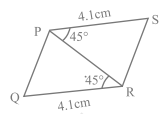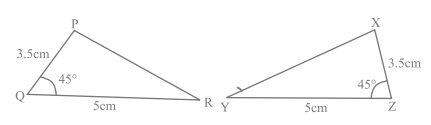# congruence of triangles class 7 worksheet

In this page we have congruence of triangles class 7 worksheets . Hope you like them and do not forget to like , social share and comment at the end of the page.

## Fill in the blanks:

Question 1.
(a) Two objects with same shape and size are said to be __________________
(b) The relation of two objects being congruent is called ____________________
(c) Two line segments are said to be congruent if they have same ________________
(d) If two angles are congruent, their _________________ are same.
(e) for the below figure$\Delta PQR \cong \Delta$ _____
(f) Two triangles are congruent, if two angles and the side included between them in one of the triangles are equal to the two angles and the side included between them of the other triangle. This is known as the _________
(g) If all three ____________ of a triangle are respectively equal to that of other triangle, the triangle may not be congruent.
(h) In congruence condition RHS, 'H' stands for _____________________
(i)Two rectangles are congruent, if they have same _____ and ______
(j) Two squares are congruent, if they have same ______

## True/false

Question 2.
(a) A circle of radius 10cm and a square of side 10cm are congruent.
(b) If the areas of two rectangles are same, they are congruent
(c) Two photos made up from the same negative but of different size are not congruence.
(d) if two sides and any angle of one triangle are equal to the corresponding sides and an angle of another triangle, then the triangles are not congruent.
(e) There is no AAA congruence criterion.
(f) Two circles having same circumference are congruent.
(g) If two triangles are equal in area, they are congruent.
(h) If two triangles are congruent, they have equal areas
. (i) AAS congruence criterion is same as ASA congruence criterion.
(j) $\Delta ABC \cong \Delta DFE$ implies $\Delta BAC \cong \Delta DEF$

## Multiple Choice Questions

Question 3.
By which of the following criterion two triangles cannot be proved congruent?
(a) AAA
(b) SSS
(c) SAS
(d) ASA

Question 4.
$\Delta ABC \cong \Delta PRQ$ and AB=5 cm, BC= 6 cm , AC=7 cm, What is the length of QR?
(a) 5 cm
(b) 6 cm
(c) 7 cm
(d) Cannot be determined

Question 5.
In the below figure , $\Delta PQR$ is congruent with the triangle(a) $\Delta XYZ$
(b) $\Delta XZY$
(c) $\Delta YZX$
(d) $\Delta ZXY$

Question 6.
If $\Delta PQR$ and $\Delta XYZ$ are congruent under the correspondence $QPR \leftrightarrow XYZ$, then which of the following is false
(a) $\angle R = \angle Z$,QR=XZ
(b) PQ=YX and PR=YZ
(c) $\angle P =\angle Y$ and QR= YZ
(d) $\angle Q = \angle X$

Question 7.
If for $\Delta ABC$ and $\Delta DEF$, the correspondence $CAB \leftrightarrow EDF$ gives a congruence, then which of the following is not true?
(a) AC = DE
(b) AB = EF
(c) $\angle A = \angle D$
(d) $\angle C = \angle E$

Question 8.
In triangles DEF and PQR, $\angle E = 80^0$, $\angle F = 30^0$, EF = 5 cm, $\angle P = 80^0$, PQ = 5 cm, $\angle R = 30^0$. By which congruence rule the triangles are congruent?
(a)SAS
(b)ASA
(c)SSS
(d) None of these

Question 9.
What is the side included between the angles M and N of $\Delta MNP$?
(a)MN
(b)NP
(c) None of these
(d) MP

## Numerical Type Questions

Question 10.
$\Delta ABC$ is isoceles with AB=AC, AD is the altitude from A to side BC,
prove that
(i) $\Delta ADB \cong \Delta ADC$
(ii) $\angle BAD= \angle CAD$

Question 11.
Prove that in an isosceles triangle, angles opposite to equal sides are equal.

Question 12.
Without drawing the triangles write all six pairs of equal measures
in each of the following pairs of congruent triangles.
(a) $\Delta STU \cong \Delta DEF$
(b) $\Delta ABC \cong \Delta LMN$
(c) $\Delta YZX \cong \Delta PQR$
(d) $\Delta XYZ \cong \Delta MLN$

Assignments